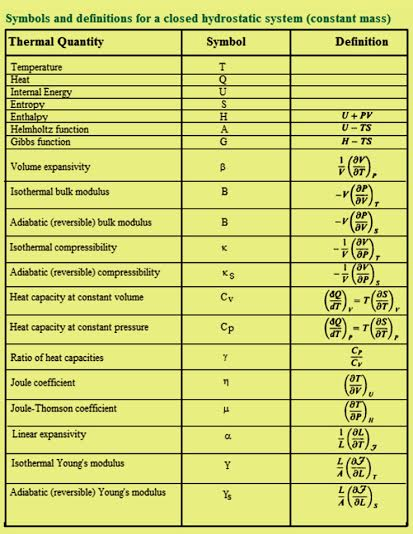# Thermodynamics Formulas

In thermodynamics, the interaction whose external system could be viewed as the raising of mass through a distance against gravitational force is defined as work done by a system on the surroundings during a process.

Thermodynamics Formulas are listed below.Some thermodynamic functions cannot be calculated directly. One needs to be able to present these quantities regarding others that can be experimentally determined.

## Solved Examples

Problem 1: Calculate how much heat is either added or removed from the system when 100kJ of work is done on a closed system during a process and the total energy of the system increases by 55.0kJ.?

By the energy conservation principle, a gross energy transfer to system effects in an equivalent increase of internal energy stockpiled in the system. This may be articulated as

Q = ΔE + W

This equation is typical statement of first law of constant mass systems. It says that in any alteration of state the heat supplied to a system is equal to the work finished by the system plus the upsurge of internal energy in the system.

Bearing in mind the work is done on a system as positive

Q + (+100.0) = +55.0

Q = +55.0 – 100.0

Q = -45.0kJ

From the result owing to the negative sign 45.0kJ of energy in heat form is removed from the system all through the procedure.

To know more examples and practice questions on thermodynamics formulas, please visit Byju’s.com